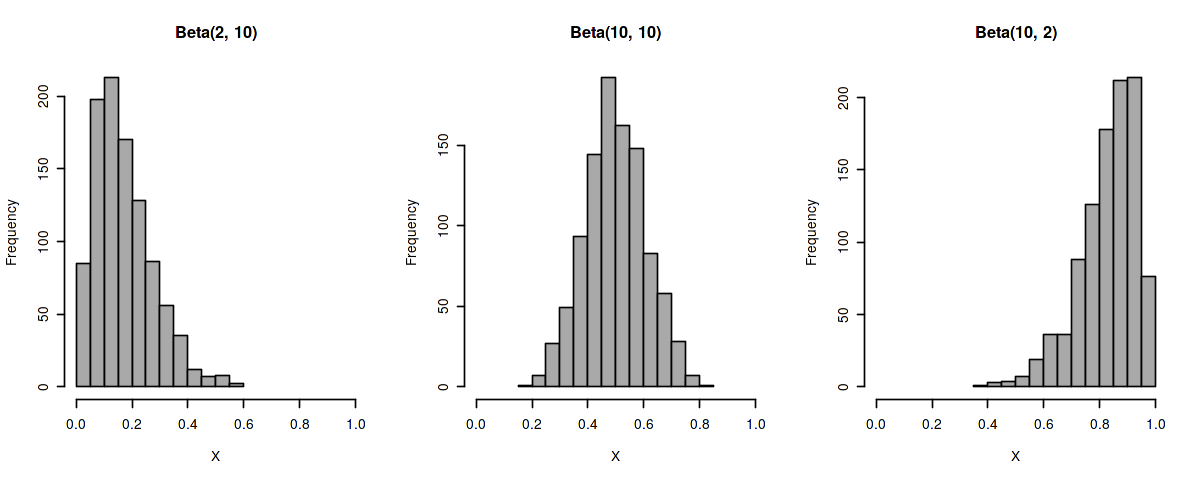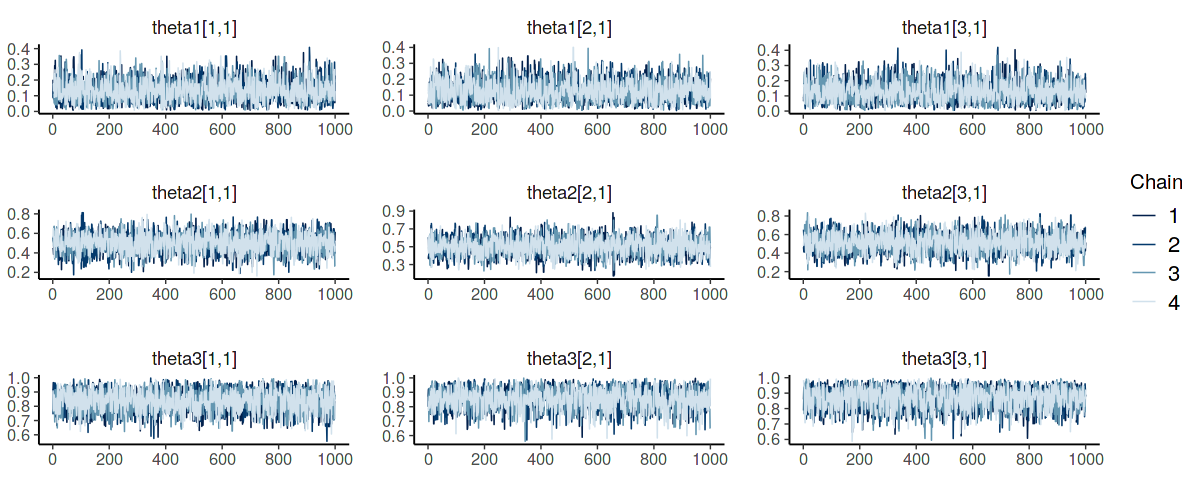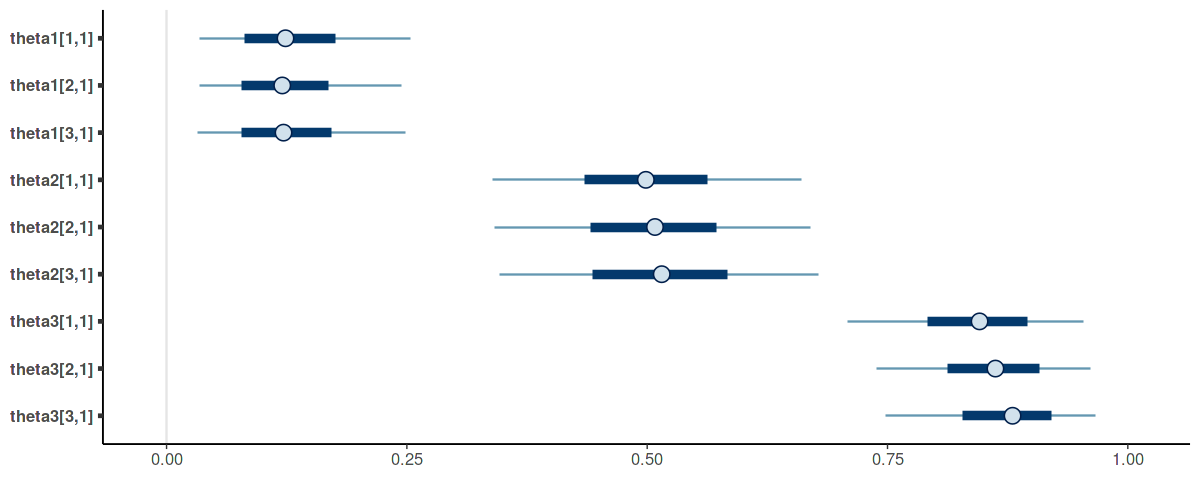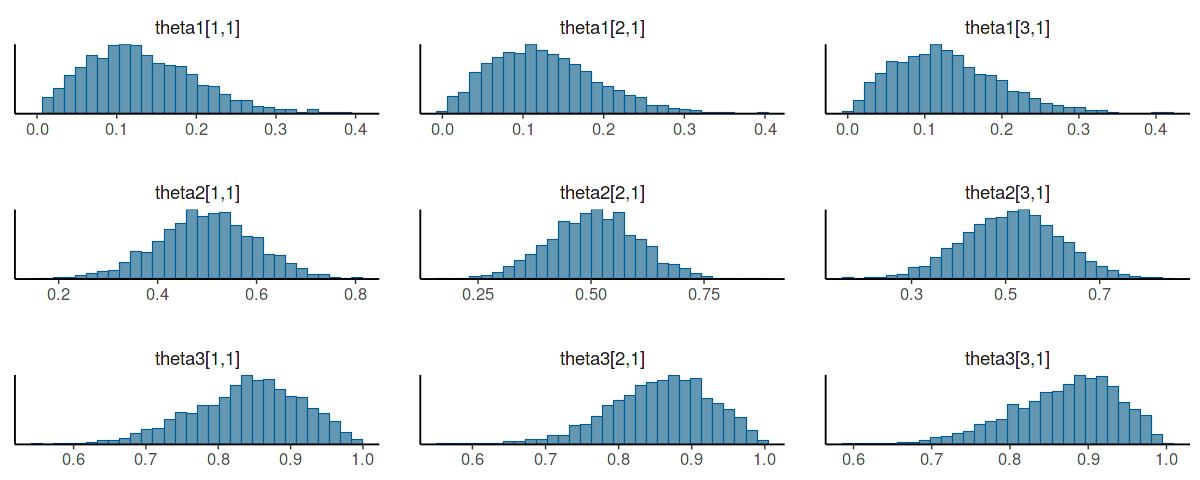# Defining custom Greta distributions¶

Greta is an R package for deep probabilistic programming and Bayesian modelling, similiar to Edward, PyMC3 or Pyro. One of Greta's key features is its easy extensibility that allows definition of custom functions that get translated and run with Tensorflow automatically. Here, I'll show how one can use Greta to define custom probability distributions for cases when Greta doesn't it already.

To setup your own distribution and do a statistical analysis with it, we first load some required libraries. To define a custom distribution, we need R6 since that is the way Greta distributions are written with. For the analysis of our model, we load bayesplot and coda for diagnostics.

In :
library(greta)
library(R6)
library(bayesplot)
library(coda)

options(repr.plot.width=10, repr.plot.height=4)

Attaching package: ‘greta’

The following objects are masked from ‘package:stats’:

binomial, cov2cor, poisson

The following objects are masked from ‘package:base’:

%*%, apply, backsolve, beta, chol2inv, colMeans, colSums, diag,
eigen, forwardsolve, gamma, identity, rowMeans, rowSums, sweep,
tapply

This is bayesplot version 1.6.0
- Online documentation and vignettes at mc-stan.org/bayesplot
- bayesplot theme set to bayesplot::theme_default()
* Does _not_ affect other ggplot2 plots
* See ?bayesplot_theme_set for details on theme setting

Attaching package: ‘coda’

The following object is masked from ‘package:greta’:

mcmc



Note that coda::mcmc masks greta::mcmc which means we should namespace the functions we use (we should do that at all times anyways).

If we have a look at a distribution that Greta is providing we see that it's basically only a couple of lines that are required. For instance, the hypergeometric distribution in R/probability_distributions.R in the Greta package looks something like this:

In :
hypergeometric_distribution <- R6Class(
"hypergeometric_distribution",
inherit = distribution_node,
public = list(

initialize = function(m, n, k, dim) {
m <- as.greta_array(m)
n <- as.greta_array(n)
k <- as.greta_array(k)
dim <- check_dims(m, n, k, target_dim = dim)
super$initialize("hypergeometric", dim, discrete = TRUE) self$add_parameter(m, "m")
self$add_parameter(n, "n") self$add_parameter(k, "k")
},

tf_distrib = function(parameters, dag) {
m <- parameters$m n <- parameters$n
k <- parameters$k log_prob <- function(x) { tf_lchoose(m, x) + tf_lchoose(n, k - x) - tf_lchoose(m + n, k) } list(log_prob = log_prob, cdf = NULL, log_cdf = NULL) }, tf_cdf_function = NULL, tf_log_cdf_function = NULL ) )  As noted above, a distribution in Greta is an R6 class that inherits from a class distribution_node, has a constructor that takes as arguments the parameters of the distribution we want to create and a dimension argument (dim). For some distributions we also have arguments like truncate when we want to truncate the distribution, for instance, to get a half-normal distribution. Within the constructor we cast the parameters using as.greta_array, check for correct dimensionality with check_dims, initialize the object, and add the parameters of the distribution as members to the object. The second method (tf_distrib) needs to define a nested function log_prob the computes the logarithm of the mass (or density) function. Note that, since Greta uses Tensorflow in the backend, we need to use functions from the tensorflow package here. The last part of the distribution class sets tf_cdf_function and tf_log_cdf_function to NULL. Having learnt how Greta defines distributions, we can try to implement one ourselves: a multivariate Bernoulli distribution, i.e. a$p$-dimensional distribution of independent Bernoullis. Since much of the functionality in the class above is private, we first define some utility functions from Greta: In : distribution_node <- .internals$nodes$node_classes$distribution_node
as.greta_array    <- .internals$greta_arrays$as.greta_array
check_dims <- .internals$utils$checks$check_dims distrib <- .internals$nodes$constructors$distrib
fl     <- .internals$utils$misc$fl tf_sum <- greta:::tf_sum  Then we define the multivariate Bernoulli (or whatever you want to call it): In : multivariate_bernoulli_distribution <- R6Class( "multivariate_bernoulli_distribution", inherit = distribution_node, public = list( initialize = function(prob, dim) { prob <- as.greta_array(prob) dim <- check_dims(prob, target_dim = dim) super$initialize("multivariate_bernoulli", dim, discrete = TRUE)
self$add_parameter(prob, "prob") }, tf_distrib = function(parameters, dag) { prob <- parameters$prob
log_prob <- function(x) {
tf_sum(x * log(prob) + (fl(1) - x) * log(fl(1) - prob))
}

list(log_prob = log_prob, cdf = NULL, log_cdf = NULL)
},

tf_cdf_function = NULL,
tf_log_cdf_function = NULL
)
)


The definition is precisely as for the hypergeometric distribution, only that we needed to change the parameters. In this case we the arguments are a $p$-dimensional vector of sucess probabilities. As for the hypergeometric distribution, we only need to set up the constructor, implement a tf_distrib function and define the log-probability. Additionally, we write a nice wrapper function that creates an object:

In :
multivariate_bernoulli <- function(prob, dim = NULL) {
distrib("multivariate_bernoulli", prob, dim)
}


That's pretty much it. Let's test our distribution with a small example. We set up a data set of 90 samples of a three-dimensional Bernoulli mixture.

In :
n <- 30
p <- 3
k <- 3
(probs <- seq(0.1, 0.9, length.out = k))

d <- rbind(
matrix(rbinom(n * p, 1, probs), n),
matrix(rbinom(n * p, 1, probs), n),
matrix(rbinom(n * p, 1, probs), n)
)

1. 0.1
2. 0.5
3. 0.9

The code above creates data from a three-component mixture model of multi-variate Bernoullis with different success probablities. Now, let's mdoel this in Greta. First we set up the priors we need. Since Greta so far does not provide an ordered keyword like Stan does, we need to make use of non-exchangable priors for the success probabilities of the single Bernoullis. Otherwise the posteriors would not be identifiable.

The Beta distribution is a natural choice for the priors. Choosing different hyperparameters let's us put most of the density differntly for each component. Since we use three components, I parameterize the priors such that the main part of the density is around $0.1$, $0.5$ and $0.9$.

The plots below show this:

In :
par(mfrow=c(1, 3))
hist(rbeta(1000, 2, 10), col = "darkgrey", xlab="X", main="Beta(2, 10)", xlim=c(0, 1))
hist(rbeta(1000, 10, 10), col = "darkgrey", xlab="X", main="Beta(10, 10)", xlim=c(0, 1))
hist(rbeta(1000, 10, 2), col = "darkgrey", xlab="X", main="Beta(10, 2)", xlim=c(0, 1))
par(mfrow=c(1, 1))Defining this in Greta is quite easy:

In :
theta1 <- greta::beta(2, 10, dim = p)
theta2 <- greta::beta(10, 10, dim = p)
theta3 <- greta::beta(10, 2, dim = p)

pi <- greta::uniform(0, 1, 3)


Then we model the distribution of every observation in our data set.

In :
for (i in seq(nrow(d)))
{
distribution(d[i,]) <- greta::mixture(
multivariate_bernoulli(theta1),
multivariate_bernoulli(theta2),
multivariate_bernoulli(theta3),
weights = pi)
}


The steps above were the ones that took us most of the time to implement while it is simple for the computer. Inferring the posteriors is easy for us, but very hard for the computer on the other hand:

In :
mod <- greta::model(theta1, theta2, theta3)
samples <- greta::mcmc(mod)

running 4 chains simultaneously on up to 4 cores

warmup ====================================== 1000/1000 | eta:  0s | 1% bad
sampling ====================================== 1000/1000 | eta:  0s


Let's check if the inference worked out nicely. First, we have a look at potential scale reduction factors to check for possible divergences.

In :
coda::gelman.diag(samples)

Potential scale reduction factors:

Point est. Upper C.I.
theta1[1,1]       1.00       1.00
theta1[2,1]       1.00       1.01
theta1[3,1]       1.00       1.00
theta2[1,1]       1.00       1.01
theta2[2,1]       1.00       1.01
theta2[3,1]       1.00       1.01
theta3[1,1]       1.00       1.01
theta3[2,1]       1.01       1.02
theta3[3,1]       1.00       1.00

Multivariate psrf

1.01

Looks ok, none of the estimates are greater than 1.05 which is usually a good indicator. In addition it always makes sense to look at the effective sample size.

In :
coda::effectiveSize(samples)

theta1[1,1]
2602.33973671931
theta1[2,1]
2414.65893630556
theta1[3,1]
2504.24586711713
theta2[1,1]
2529.47224443831
theta2[2,1]
2766.63949634105
theta2[3,1]
2757.4459721625
theta3[1,1]
2208.28249641005
theta3[2,1]
2351.80168330971
theta3[3,1]
2535.30094514505

Finally, we should have a look at the posterior traces to see if some chain went wrong.

In :
bayesplot::mcmc_trace(samples)The three diagnostics above pose a great way to check your model and if something went wrong. Often, if, for instance, the psrfs are high or effective sample size is very low, we might have poorly designed our model and need to put more thought into constructing priors or simplifying parts of the model.

Finally let's look at the posteriors and if we could infer the parameters, i.e. the success probabilities.

In :
bayesplot::mcmc_intervals(samples)In :
bayesplot::mcmc_hist(samples)

stat_bin() using bins = 30. Pick better value with binwidth.